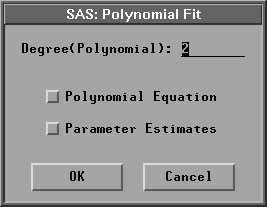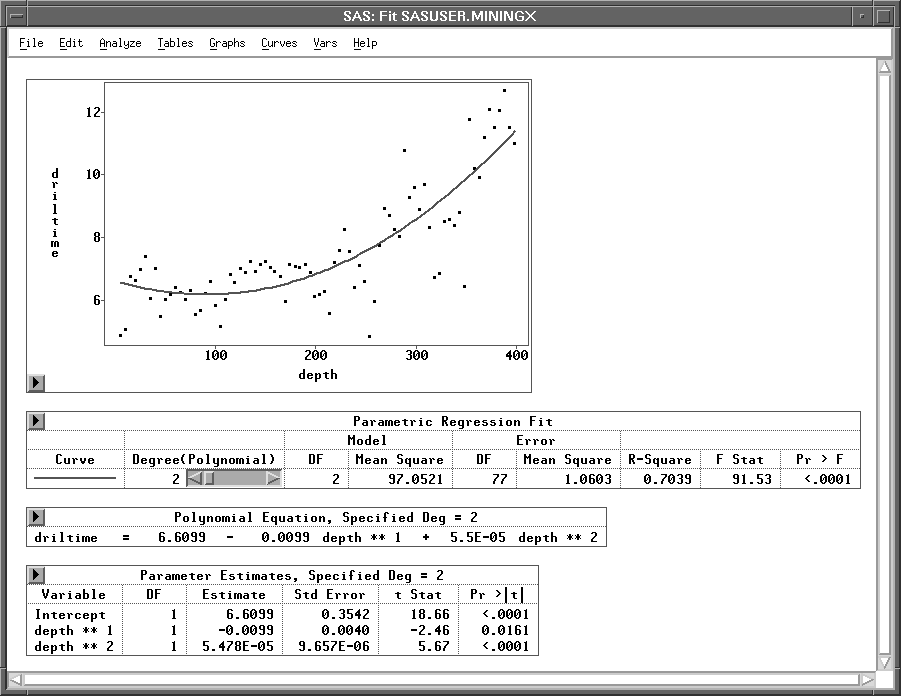Fit Analyses

## Parametric Curves: Polynomial

Choose Curves:Polynomial from the menu to add a polynomial regression fit to the Y-by-X scatter plot. This displays the Polynomial Fit dialog in Figure 39.36.Figure 39.36: Polynomial Fit Dialog

In the Polynomial Fit dialog, you enter the degree for the polynomial fit. Select the Polynomial Equation or Parameter Estimates options to create a Polynomial Equation or Parameter Estimates table for the fitted curve.

Information about the polynomial fit is displayed in a table, as illustrated by Figure 39.37 The information includes the R2 value and an F statistic and its associated p-value for testing the null hypothesis that all parameters are 0 except for the intercept. A parametric regression fit table includes the following:

Curve
is the curve in the Y-by-X scatter plot.

Degree(Polynomial)
is the degree for the polynomial fit.

Model DF
is the degrees of freedom for model.

Model Mean Square
is the mean square for model.

Error DF
is the degrees of freedom for error.

Error Mean Square
is the mean square for error.

R-Square
is the proportion of the (corrected) total variation attributed to the fit.

F Stat
is the F statistic for testing the null hypothesis that all parameters are zero except for the intercept. This is formed by dividing the mean square for model by the mean square for error.

Pr > F
is the probability under the null hypothesis of obtaining a greater F statistic than that observed.

Figure 39.37 displays a quadratic polynomial fit with Polynomial Equation and Parameter Estimates tables.Figure 39.37: A Quadratic Polynomial Fit

You can use the Degree(Polynomial) slider in the Parametric Regression Fit table to change the degree of the polynomial curve fit. However, these will not change the Polynomial Equation and Parameter Estimates tables. You can produce a new Polynomial Equation or Parameter Estimates table by selecting the Polynomial Equation or Parameter Estimates option from the Polynomial Fit dialog.